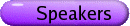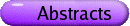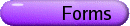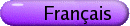Combinatorics and Geometry Org: Ian Goulden (Waterloo)[PDF] FRANÇOIS BERGERON, UQAM Diagonal coinvariant space, what's up? [PDF] We will give a description of some of the current developments surrounding the study of diagonal coinvariant space, for reflection groups, and related spaces. NANTEL BERGERON, York University Lattices, Representations and (Hopf) Algebras [PDF] We show that we can associate two graded dual structures of bialgebra to the Grothendieck ring associated to certain tower of lattices. This is work in progress with M. Zabrocki and H. Thomas. GRAHAM DENHAM, University of Western Ontario Discrete Morse theory for infinite complexes [PDF] Ten years after its introduction, discrete Morse theory has proven to be a wonderful tool to study the homotopy type of a finite, combinatorially defined complex. I will describe a generalization to a class of infinite complexes: as an example, one obtains new proofs that certain combinatorial models for classifying spaces are, in fact, aspherical. JOHN IRVING, Wilfrid Laurier University Tree Pruning and Transitive Factorizations in the Symmetric Group [PDF] The primary focus of this talk will be the (genus 0) Hurwitz enumeration problem, which asks for the number H0(p) of decompositions of a given permutation p into an ordered product of a minimal number of transpositions such that these factors act transitively on the underlying set of symbols. (The problem is typically phrased in terms of counting almost simple branched coverings of the sphere by the sphere with arbitrary ramification over one special point, but the two phrasings are equivalent.) I shall demonstrate that transitive factorizations can be encoded as planar edge-labelled maps with certain descent structure, and describe a bijection that "prunes trees" from such maps. This allows for a shift in focus from the combinatorics of factorizations to the sometimes more manageable combinatorics of smooth maps. As a result, we gain some combinatorial insight into the nature of Hurwitz's famous formula for H0(p), and derive new bijections that prove his formula in certain restricted cases. DAVID JACKSON, University of Waterloo, 200 University Avenue West, Waterloo, Ontario N2L 3G1 Combinatorial aspects of the double Hurwitz numbers and Faber's intersection numbers [PDF] Faber's intersection numbers, double Hurwitz numbers and Hurwitz numbers contain a surprising amount of combinatorial structure. Goulden, Vakil and I hope to gain further understanding of the Faber intersection numbers by examining their relationship to the double Hurwitz numbers. I shall give an account of the high points of this work by starting with the classical case of genus zero covers of the sphere, for it is in this case that there is a glimpse of an instance of a basic algebraic-combinatorial structure that appears to pervade our approach. Vakil's earlier talk has given an introduction to the moduli space of curves and an impression of how such geometric questions may be translated into combinatorics. I shall describe how an approach through algebraic combinatorics may be used to determine further properties of these numbers. JOEL KAMNITZER, University of California, Berkeley Mirkovic-Vilonen polytopes [PDF] We will discuss the Mirkovic-Vilonen polytopes which give a new model for the combinatorics of representations of complex reductive groups. These polytopes are the moment map images of the Mirkovic-Vilonen cycles, which are varieties arising from the geometric Satake Isomorphism. They also arise from the combinatorics of Lusztig's canonical basis. We will discuss new results concerning a crystal structure on the set of these polytopes. ALLEN KNUTSON, UC Berkeley Multidegrees, their computation, and applications [PDF] Homogeneous polynomials can be interpreted as T-equivariant cohomology classes on affine space. Having positive coefficients is a sign that they are geometric, or more precisely, "effective", being representable as the class of a T-invariant subscheme. With such a geometric interpretation in hand, there are various ways to compute the class in automatically positive ways. I'll explain a couple of general recipes for doing this, one being "geometric vertex decompositions", and apply them to matrix Schubert varieties; one payoff will be a bunch of old and new formulæ for double Schubert (and Grothendieck) polynomials. It's not too much of a surprise, though, that geometry helps one compute Schubert polynomials, as they have a geometric origin. So I'll also talk about a very surprising application of multidegrees in statistical mechanics, where the combinatorics predated the geometry, and is still very mysterious. This work is joint with Ezra Miller, Alex Yong, and Paul Zinn-Justin. KEVIN PURBHOO, University of British Columbia The generalised Horn recursion [PDF] The classical statement of Horn's conjecture gives a recursively defined set of inequalities for possible eigenvalues of triples of Hermitian matrices (A,B,C) satisfying A+B+C=0. Reformulated, however, this recursion tells us that there is a recursive nature to the set of non-vanishing Littlewood-Richardson numbers. I'll discuss a generalisation which recursively characterises the non-vanishing Schubert intersection numbers for all minuscule flag varieties. This is joint work with Frank Sottile. KONSTANZE RIETSCH, King's College, London (UK), and Waterloo Quantum cohomology of G/P and the Peterson variety [PDF] Let G be a reductive linear algebraic group. The Peterson variety is a projective subvariety of the Langlands dual flag variety which, by a remarkable theorem of Dale Peterson's, has strata that are isomorphic to Spec(qH*(G/P)) for the varying parabolic subgroups P in G. We give a new construction of these strata shedding some (vague) light on the why and wherefore of Peterson's theorem. MIKE ROTH, Queen's University, Kingston, Ontario Varieties with positive definite intersection form [PDF] The talk will discuss the problem of finding varieties with positive definite middle intersection form. MISHA SHAPIRO, Michigan State University Single and double Hurwitz numbers [PDF] The classical problem of finding numbers of ramified covers of the two dimensional sphere of fixed ramification types goes back to Hurwitz. The interests to Hurwitz numbers was revived after physicists discovered that these numbers play central role in quantum chromodynamics. I will describe the method of computing so-called single Hurwitz numbers. These formulas were used by Okounkov and Pandharipande to give another proof of Witten's conjecture. We will also discuss an approach to Hurwitz numbers of coverings with two non-simple branchings. GREG SMITH, Queen's Unversity Toric varieties as fine moduli spaces [PDF] We'll discuss how to construct projective simplicial toric varieties as the fine moduli space of representations of a quiver. HUGH THOMAS, University of New Brunswick The lattice of noncrossing partitions via representation theory of quivers [PDF] Associated to any finite reflection group, there is a combinatorial object called the lattice of noncrossing partitions. In type A, these are just the classical noncrossing partitions. In this talk, I will discuss a new approach to the lattice of noncrossing partitions for crystallographic reflection groups, using the representation theory of quivers. This approach yields a new proof that the noncrossing partitions do indeed form lattices for these groups (a result proved in a type-free way for the first time earlier this year by Brady and Watt), and also clarifies connections between noncrossing partitions, clusters, and other related objects. This is joint work with Colin Ingalls. RAVI VAKIL, Stanford University, Stanford, CA 94305, USA Double Hurwitz numbers and the intersection theory of the moduli spaceof curves [PDF] The moduli space of genus g curves, the "parameter space" of all genus g Riemann surfaces, is a central object in geometry. Its topology exhibits some remarkable structure. As an important example, Faber's "intersection number conjecture" predicts that its cohomology ring exhibits unsuspected combinatorial structure, related to the symmetric group. This conjecture will soon be a theorem, thanks to Givental's proof of the "Virasoro conjecture for projective space" and the details soon to be provided in a book by Lee and Pandharipande. However, this proof does not "explain" why this combinatorial structure should be there. Ian Goulden, David Jackson, and I hope to do this by exploiting a relationship of these intersection numbers with double Hurwitz numbers; this programme is complete in a large class of cases ("up to three points"). In this lecture I will give an introduction to the moduli space of curves and Faber's conjecture, and I will give some impression of how to translate these geometric questions into combinatorics. The translation uses joint work with Tom Graber. ALEXANDER YONG, UC Berkeley/Fields Institute On Smoothness and Gorensteinness of Schubert varieties [PDF] The study of singularities of Schubert varieties in the flag manifold involves interesting interplay between algebraic geometry, representation theory and combinatorics. Although all Schubert varieties are Cohen-Macaulay, few are smooth. An explicit combinatorial characterization of the smooth ones was given by Lakshmibai and Sandhya (1990). The singular locus of an arbitrary Schubert variety was determined around 2001 by several authors. Gorensteinness is a measurement of the "pathology" of the singularities of an algebraic variety; it logically sits between smoothness and Cohen-Macaulayness. We explicitly characterize which Schubert varieties are Gorenstein, analogous to Lakshmibai and Sandhya's theorem. Here is the geometric interpretation: a Schubert variety is Gorenstein if and only if it is Gorenstein at the generic points of the singular locus. We also compute the canonical sheaf of a Gorenstein Schubert variety as a line bundle in terms of the Borel-Weil construction. I will discuss the geometric corollaries and questions that arise in this work. This is a joint project with Alexander Woo.